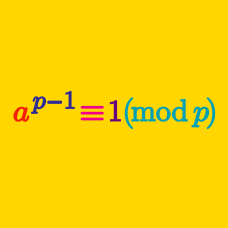Number Theory

# Modular Arithmetic - Word Problems

There are $$81$$ groups of $$21$$ students. When we regroup all of the students so that each group has $$5$$ members, how many students will be left without a group?

John and Amy have $$48$$ and $$51$$ quarters, respectively. If they combine all of their quarters and change as much as possible into dollar bills, how many quarters will be left?

Note: There are 4 quarters in a dollar.

November and December have $$30$$ and $$31$$ days, respectively. If November $$1$$ is the first day of the week, how many days of December are there in the last week?

How many different values are there for $$4 ^ n \pmod { 7}$$, as $$n$$ ranges over all positive integers?

What is the sum of all positive prime numbers $$x$$ such that $$x^2+2$$ is also a prime?

×